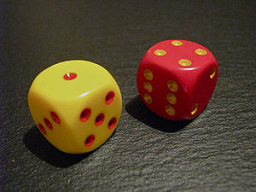Three dices

What is the probability that the sum of points 14 will be a roll of three dice (B, M, Z)?

p =  0.0694

Step-by-step explanation:Did you find an error or inaccuracy? Feel free to write us. Thank you!Tips to related online calculators
Do you solve Diofant problems and looking for a calculator of Diofant integer equations?